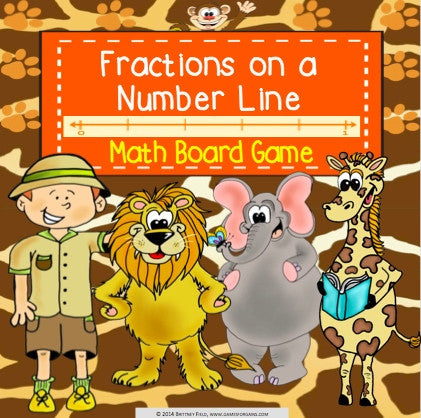## Fractions on a Number Line Board Game

• \$325

Fractions on a Number Line Board Game contains 30 WORD PROBLEM game cards and a game board to help students practice recognizing fractions and whole number fractions on a number line (3.NF.A.2, 3.NF.A.2.A, 3.NF.A.2.B, 3.NF.A.3.C). This game works great as a pair/group activity, or for use in math centers.

Included in this Fractions on a Number Line Board Game:
Instructions for play
Game board
30 word problem game cards, addressing the following skills:
*Naming the fractions between 0 and 1 on a number line when partitioned into equal parts
*Naming the fraction at a specific point between 0 and 1 on a number line
*Drawing a number line showing fraction intervals to represent a word problem
*Naming fractions greater than 1 on a number line

The answers are included on the back of the cards.